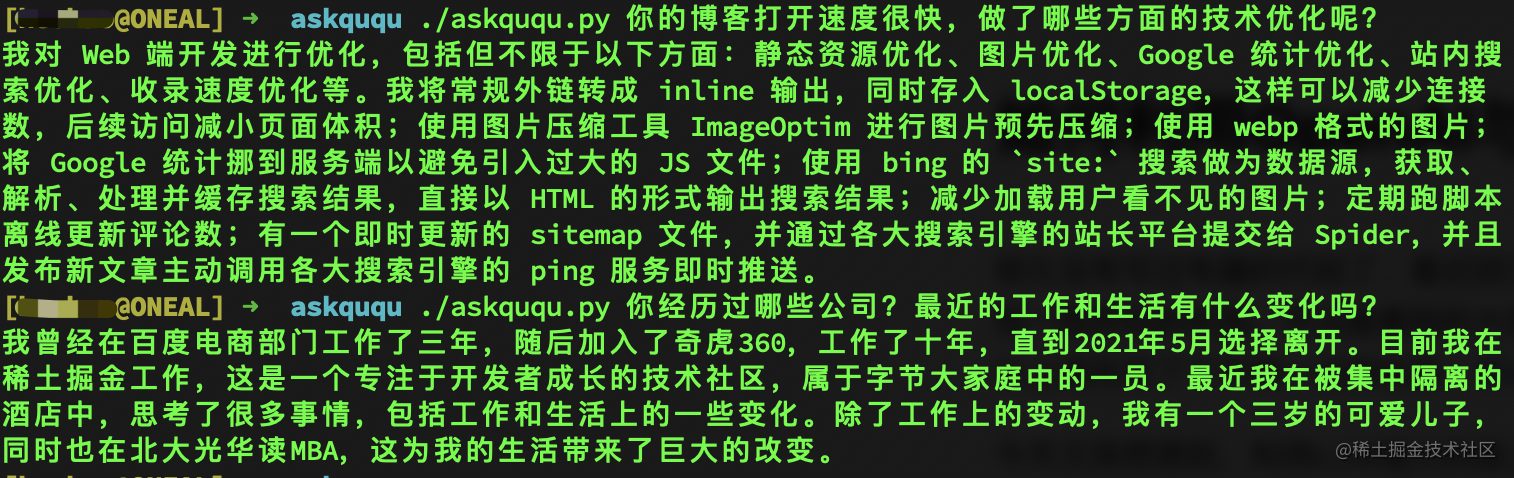## 实现原理

TL;DR: 先将文章内容通过 OpenAI 的 Embedding 模型转化成向量数据，然后与问题进行向量距离计算，最后将topK答案扔给 OpenAI 润色输出。

### 第二步，优化内容token长度

``````import os
import tiktoken

tokenizer = tiktoken.get_encoding("cl100k_base")
posts = []

# 假如我们第一步先将文章内容保存到了本地./posts 目录下
for file in os.listdir('posts/'):
with open('posts/' + file, 'r', encoding='UTF-8') as f:
[title, content] = text.split('@@@')
tokens = len(tokenizer.encode(content))
posts.append({'title': title, 'content': content, 'tokens': tokens})``````

tiktoken 是 OpenAI 提供的一个工具，可以快速标记文本成模型 token 以计算其长度。

``````def split_text(text, max_tokens = max_tokens):
sentences = text.split('。') #按句号分割，以防将同一个句子分割到了不同的text断中
n_tokens = [len(tokenizer.encode(" " + sentence)) for sentence in sentences]
chunks = []
tokens_so_far = 0
chunk = []

for sentence, token in zip(sentences, n_tokens):
if tokens_so_far + token > max_tokens:
chunks.append("。".join(chunk) + "。")
chunk = []
tokens_so_far = 0
if token > max_tokens:
continue

chunk.append(sentence)
tokens_so_far += token + 1
return chunks

ebd_posts = []
for post in posts:
if post['content'] is None:
continue
if post['tokens'] > max_tokens:
splited_posts = split_text(post['content'])
for _post in splited_posts:
ebd_posts.append((post['title'], _post))
else:
ebd_posts.append((post['title'], post['content']))``````

### 第三步，打标

``````import pandas as pd
import openai

df = pd.DataFrame(ebd_posts, columns = ['title', 'content'])
df['tokens'] = df.content.apply(lambda x: len(tokenizer.encode(x)))

# 以下这行代码会多次请求 OpenAI 接口，会有较长的等待时间，如果数据量大可以自行做优化处理
df['embeddings'] = df.content.apply(lambda x: openai.Embedding.create(input=x, engine='text-embedding-ada-002')['data']['embedding'])

df.to_csv('data/embeddings.csv')

### 第四步，计算匹配内容

``````import pandas as pd
import numpy as np
import openai
from openai.embeddings_utils import distances_from_embeddings

max_len = 4000 #4096 for gpt-3.5-turbo

df['embeddings'] = df['embeddings'].apply(eval).apply(np.array)

def match_text(question):
context = []
cur_len = 0

# 问题打标

# 使用 OpenAI 提供的工具函数做向量匹配，如果是存储的向量数据库，查询计算更方便
df['distances'] = distances_from_embeddings(q_embeddings, df['embeddings'].values, distance_metric='cosine')
for i, row in df.sort_values('distances', ascending=True).iterrows():
cur_len += row['tokens']
if cur_len > max_len:
break
context.append(row['content'])
return context``````

### 第五步，优化回答内容

``````import openai

def q(question=''):

try:
# prompt 可以自己调整优化，其他参数也可以根据需要修改
completion = openai.ChatCompletion.create(
model='gpt-3.5-turbo',
messages=[
{'role': 'system', 'content': f"你叫xxx，是一个热心和大家分享和交流的人，你擅长的领域有xxxx。"},
{'role': 'user', 'content': f"根据context提供的信息回答我提出的问题。\n\nContext: {context}\n\n---\n\nQuestion: {question}\nAnswer:"}
]
)
return completion['choices']['message']['content']
except Exception as e:
print(e)
return ''``````